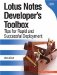# What Is a Formula?

In the simplest terms, formulas are used to produce a data value or action. This value or action is derived by using one or more commands, functions, or statements to create a main expression. All formulas must have a main expression that equates to a result. If the formula does not produce a value or action, the Designer client will not allow you to save the formula.

For example, the following illustrates an invalid formula. Here, the text value "Happy New Year" is assigned to a temporary variable called message. Although the formula does perform a calculation, there is no main expression. This formula does not produce a result.

```message := "Happy New Year";
```

For this example to be valid, the formula must do something to produce a result. This second example illustrates a valid formula. Looking at this formula, you'll notice that the first line performs a computation by assigning a text value to a temporary variable. The statement is terminated with a semicolon, which separates the first statement from the second. The formula then ends with the main expression, which defines the value for the field.

```message := "Happy New Year";
message
```

In this case, the main expression is assigned the contents of the variable message. Alternatively, the same result could have been achieved by setting the text string to be the main expression, as illustrated next.

```"Happy New Year"
```

Tip

When writing a formula containing multiple statements, each statement must be separated by a semicolon, and the last line in the formula must be the "main expression."Lotus Notes Developers Toolbox: Tips for Rapid and Successful Deployment
ISBN: 0132214482
EAN: 2147483647
Year: N/A
Pages: 293
Authors: Mark Elliott